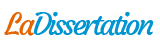# Dynamique des fluides en temps réel pour les jeux (document en anglais)

Note de Recherches : Dynamique des fluides en temps réel pour les jeux (document en anglais). Recherche parmi 256 000+ dissertations

Par   •  1 Octobre 2014  •  4 927 Mots (20 Pages)  •  493 Vues

Page 1 sur 20

Real-Time Fluid Dynamics for Games

Jos Stam

Alias | wavefront

210 King Street East

Email: jstam@aw.sgi.com,

Url: http://www.dgp.toronto.edu/people/stam/reality/index.html.

Abstract

In this paper we present a simple and rapid implementation of a fluid dynamics solver for game

engines. Our tools can greatly enhance games by providing realistic fluid-like effects such as

swirling smoke past a moving character. The potential applications are endless. Our algorithms

are based on the physical equations of fluid flow, namely the Navier-Stokes equations. These

equations are notoriously hard to solve when strict physical accuracy is of prime importance.

Our solvers on the other hand are geared towards visual quality. Our emphasis is on stability

and speed, which means that our simulations can be advanced with arbitrary time steps. We

also demonstrate that our solvers are easy to code by providing a complete C code

implementation in this paper. Our algorithms run in real-time for reasonable grid sizes in both

two and three dimensions on standard PC hardware, as demonstrated during the presentation

of this paper at the conference.

Introduction

Fluid flows are everywhere: from rising smoke, clouds and mist to the flow of rivers and

oceans. Because one of the major goals of games is to immerse players into plausible virtual

worlds, it is desirable to include fluid flows into game engines. There already exist many ad-

hoc models that attempt to fake fluid-like effects, such as particles rendered as textured

sprites. However, animating them in a convincing manner is not easy.

We believe that a better alternative is to use the physics of fluid flows which have been

developed since the time of Euler, Navier and Stokes (from the 1750’s to the 1850’s). These

developments have led to the so-called Navier-Stokes Equations, a precise mathematical

model for most fluid flows occurring in Nature. These equations, however, only admit analytical

solutions in very simple cases. No progress was therefore made until the 1950’s when

researchers started to use computers and develop numerical algorithms to solve the

equations. In general, these algorithms strive for accuracy and are fairly complex and time

consuming. This is because the applications that require these solvers have to be physically

accurate. It is obviously crucial that the stresses and drag on an airplane or a bridge are

calculated precisely.

In computer graphics and in games on the other hand what matters most is that the

simulations both look convincing and are fast. In addition it is important that the solvers aren’ttoo complex so that they can be implemented on standard PCs, game consoles or PDAs. In

this paper we present a set of algorithms which meet these requirements. To achieve these

goals we depart from conventional wisdom in computational physics and develop algorithms

custom tailored for creating visual effects. Unlike physically accurate solvers which have strict

bounds on their time steps, our algorithms are stable, and never “blow up.”

The Physics of Fluids

Figure 1: The Navier-Stokes Equations for the velocity in a compact vector notation (top) and

the equation for a density moving through the velocity field (bottom).

Mathematically, the state of a fluid at a given instant of time is modeled as a velocity vector

field: a function that assigns a velocity vector to every point in space. Imagine the air that

occupies a room, its velocity will vary due to the presence of heat sources, air drafts, etc. For

example, the velocity of the air near a radiator will predominantly be pointing in the upward

direction due to heat rising. The distribution of velocities within a room is also quite complex as

is evident when watching the smoke rising from a cigarette or the motion of dust particles in

the air.The Navier-Stokes Equations are a precise description of the evolution of a velocity field

over time. Given the current state of the velocity and a current set of forces, the equations tell

us precisely how the velocity will change over an infinitesimal time step. Figure 1 (top) depicts

these equations in a compact vector-like notation. Very roughly the equation states that the

change in velocity is due to the three terms on the right hand side of the equal sign.

A velocity field on its own isn’t really visually interesting until it starts moving objects such as

smoke particles, dust or leaves. The motion of these objects is computed by converting the

velocities surrounding the object into body forces. Light objects such as dust are usually just

carried along with the velocity field: they simply follow the velocity. In the case of smoke, it is

prohibitively expensive to model every particle. Hence in this case the smoke particles are

...

Télécharger au format  txt (32 Kb)   pdf (271.9 Kb)   docx (25.5 Kb)
Voir 19 pages de plus »